# Sort Name by Last Name in Excel

This post will guide you how to sort full name by last name in excel. How to sort full names by last name using Text to Columns command in excel. How do I sort names by last name with excel formula.

## Sort Names by Last Name using Excel Formula

Assuming that you have a list of names in your worksheet and you would like to alphabetize by last name. You can create an excel formula based on the RIGHT function, the LEN function, the FIND function and the SUBSTITUTE function.

You need to extract the middle name firstly from the full names, then you can use the sort command to sort the Last names. Then the columns with full name should be sorted by Last name.

Let’s see the following example to sort full names in range B2:B4 by last name.

1# you can write down the following formula to extract last name:

`=RIGHT(B2, LEN(B2)- FIND("#",SUBSTITUTE(B2," ","#",2),1))`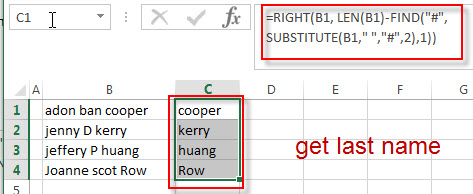If you want to know how this formula works, you can refer to this post: How to get First name, Middle name and Last name.

2# select the cells that contain last name in range C1:C4, and then click “DATA”-> Sort A to Z.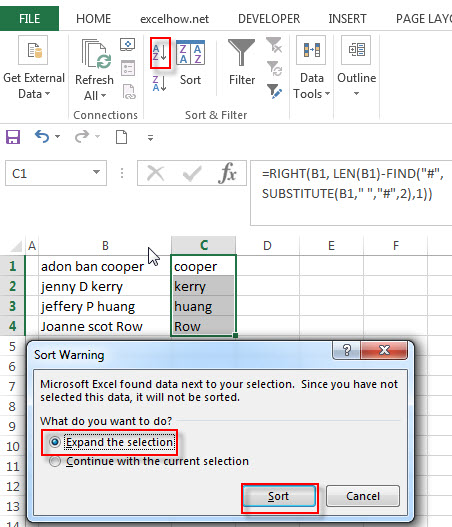3# select “Expand the selection” radio button. Then click “Sort” button. You will see that the full names have been sorted by last names.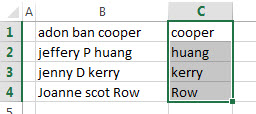## Sort Names by Last Name using Text to Columns command

You can also use the Text to Columns command to extract the Last name from a full name in excel. Then you can sort the full name by Last name cells. Just refer to the following steps:

1# Select the range of cells that contains full name, then click on Data -> Text to Columns (under Data Tools group)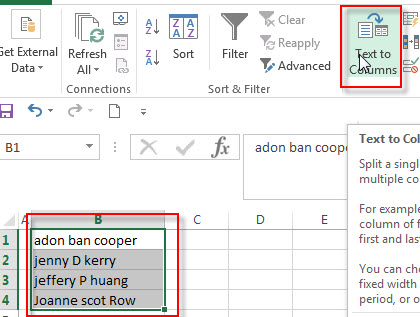2# the “Convert Text to Columns Wizard” windows will appear. Then select Delimited radio button. Click “Next” button.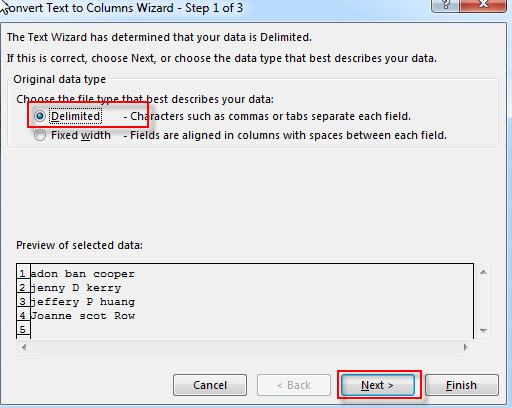3# select Space option in Delimiters section box, then click “Next” button.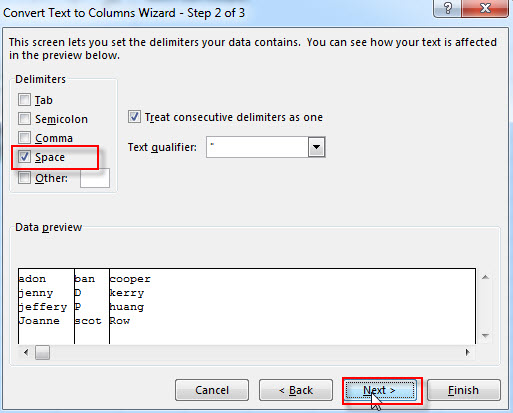4# choose Destination Cell, such as: C1, then click “Finish” button.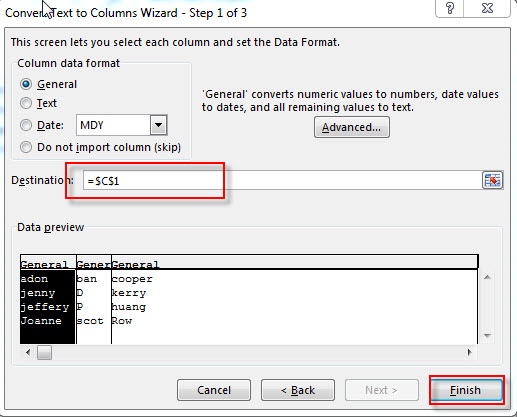5# you will see that all full names have been split into three columns.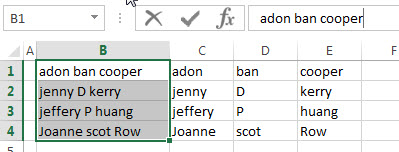6# select cells that contain last name, then click Data-> Sort A to Z command, choose “Expand the selection” radio button, then click “sort” button.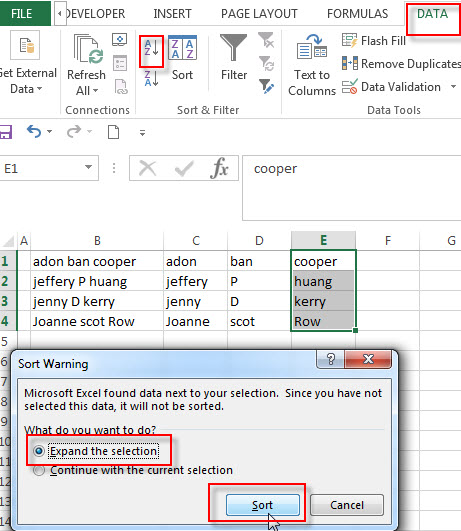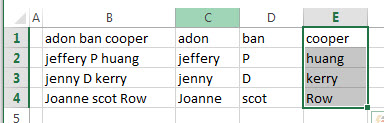### Related Posts

• Split full name to first and last name
You can create another formula based on the RIGHT function, the LEN function, the FIND function and the SUBSTITUTE function to extract the last name……
• Sort Names by Middle Name in Excel
Assuming that you have a list of names in your worksheet and you would like to alphabetize by middle name. You can create an excel formula based on the IF function, the ISERR function, the FIND function, and the MID function.…
• Get first word from text string
If you want to extract the first word from a text string in a cell, you can use a combination of the IF function, the ISERR function, the LEFT function and the FIND function to create a complex excel formula..…
• Get last word from text string
If you want to get the last word from a text string, you can create an excel formula based on the RIGHT function, the LEN function, the FIND function and the SUBSTITUTE function..…
• Extract nth word from text string
If you want to extract the nth word from a text string in a single cell, you can create an excel formula based on the TRIM function, the MID function, the SUBSTITUTE function, the REPT function and the LEN function..…

### Related Functions

• Excel FIND function
The Excel FIND function returns the position of the first text string (sub string) within another text string.The syntax of the FIND function is as below:= FIND(find_text, within_text,[start_num])…
• Excel RIGHT function
The Excel RIGHT function returns a substring (a specified number of the characters) from a text string, starting from the rightmost character.The syntax of the RIGHT function is as below:= RIGHT (text,[num_chars])…
• Excel Substitute function
The Excel SUBSTITUTE function replaces a new text string for an old text string in a text string.The syntax of the SUBSTITUTE function is as below:= SUBSTITUTE  (text, old_text, new_text,[instance_num])….
• Excel LEN function
The Excel LEN function returns the length of a text string (the number of characters in a text string).The syntax of the LEN function is as below:= LEN(text)…